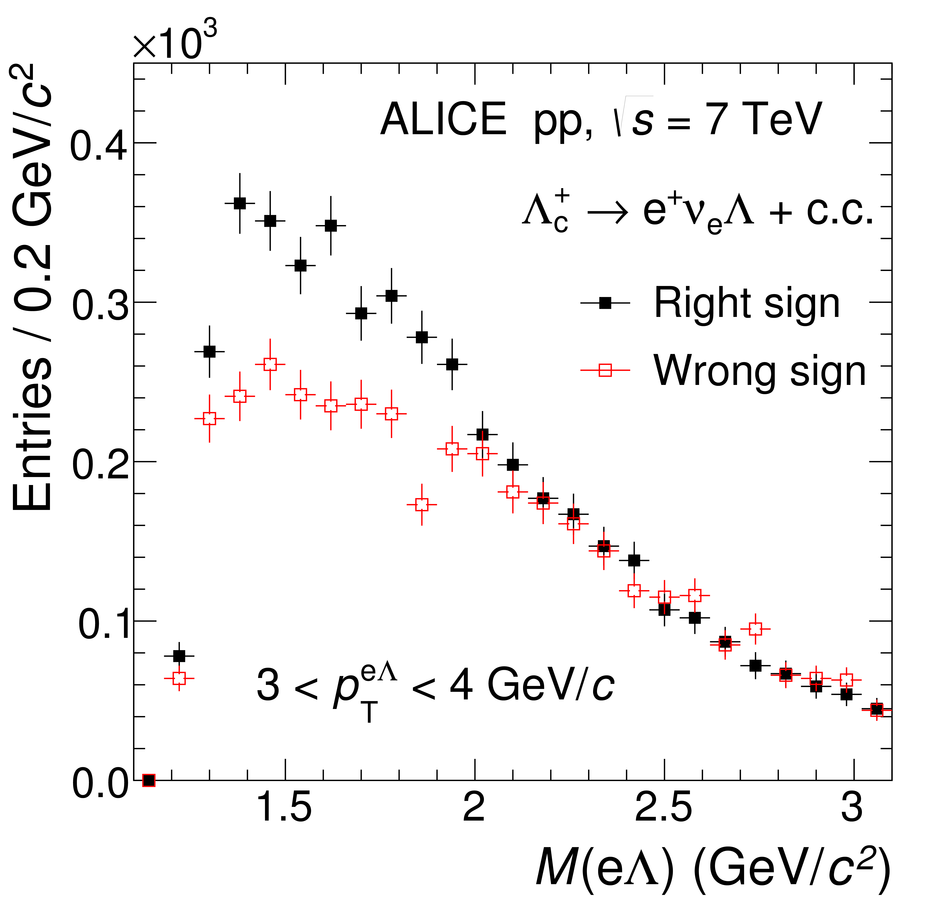# Figure 5

 Invariant mass distributions of e$\Lambda$ pairs for RS and WS combinations in the interval $3 < \pt^{{\rm e}\Lambda} < 4$ \gevc in pp collisions at $\sqrt{s}$~= 7 TeV.